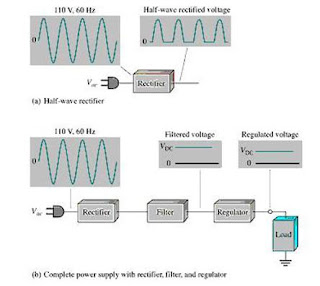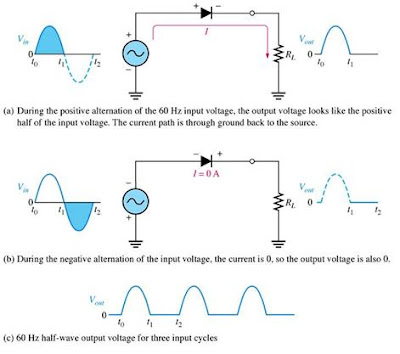# Half Wave Rectifiers Theory and Circuit Operation

Half Wave Rectifiers Theory and Circuit Operation:

In order to convert an AC voltage into DC voltage, rectifiers are used  In all power supplies that operate from an ac voltage source, rectifiers are used

1) Basic Power Supply:

The power supply converts the standard 110 Vac (AC voltage) into a constant dc voltage. All electronic devices such as (TVs, VCRs, DVDs, etc) have at least one rectifier circuit. A rectifier circuit is used to convert an ac input voltage to a pulsating dc voltage. The filter circuit can eliminates the fluctuations in the rectified output voltage. The regulator circuit is used for maintaining a constant dc voltage for various inputs and load resistances. The circuit (or device) receiving power from the source s called as the load .Figure: Block diagram of a rectifier ciruit and a dc power supply with a load
a) Half-Wave Rectifier :

The half-wave rectification process is illustrated below.Figure: Operation of a half-wave rectifier circuit
–The diode is forward biased, when the sinusoidal input (Vin) goes positive thus the diode conducts current on the positive half cycle of the input voltage. This will makes the output voltage keeps as the shape of the input voltage.

– When the input voltage (Vin ) becomes negative (second half of cycle), the diode is reverse biased. (This is due to the condition that in order to make a diode as forward biased condition; a higher voltage should be applied at the positive side (P side) of the diode compared to the negative part of the diode. In other words if a higher voltage is applied at the P side of the diode, it conducts).

When the diode is in the reverse biased state, there is no current.

AT this time, the voltage across the load resistor RL is 0V.

SO the net result is a pulsating dc voltage with the same frequency as the input voltage.

The average value of the pulsating wave is

VAVG = Vp/p
Since the cut off voltage for a silicon diode is 0.7 Volts, we also have to take the 0.7 V from the barrier potential into account.

Thus we should get:
Vp(out) = Vp(in) – 0.7 V

a) Peak Inverse Voltage (PIV):  PIV equals the peak value of the input voltage. In other words PIV can be defined as the maximum reverse voltage that can be with stand by the diode. After the PIV voltage, the diode will get damaged or (breakdown condition occurs after PIV).

A diode must be able to withstand this amount of the applied repetitive reverse voltage.

PIV = Vp(in)